# Constructal Design Applied to the Study of the Geometry and Submergence of an Oscillating Water Column

Constructal Design Applied to the Study of the Geometry and Submergence of an Oscillating Water Column

Giulio Lorenzini* Maria Fernanda Espinel Lara Luiz Alberto Oliveira Rocha Mateus das Neves Gomes Elizaldo Domingues dos Santos Liércio André Isoldi

Dipartimento di Ingegneria Industriale, Università degli Studi di Parma, Parco Area delle Scienze 181/A, 43124 Parma, Italy, giulio.

Departamento de Engenharia Mecânica, Universidade Federal do Rio Grande do Sul, Rua Sarmento Leite, 425, Porto Alegre, RS, 90.050-170, Brazil;

Instituto Federal do Paraná, Campus Paranaguá, Rua Antônio Carlos Rodrigues 453, bairro Porto Seguro, Paranaguá, PR, 83215-750, Brazil;

School of Engineering, Universidade Federal do Rio Grande, Av. Itália Km 8, bairro Carreiros, Rio Grande, RS, 96201-900, Brazil.

Corresponding Author Email:
giulio.lorenzini@unipr.it; giulio.lorenzini@corresponding;maria.lara@ufrgs.br;luizrocha@mecanica.ufrgs.br;mateus.gomes@ifpr.br;elizaldosantos@furg.br, liercioisoldi@furg.br
Page:
31-38
|
DOI:
https://doi.org/10.18280/ijht.330205
N/A
|
Accepted:
N/A
|
Published:
30 June 2015
| Citation

OPEN ACCESS

Abstract:

The wave energy conversion into electricity has been increasingly studied in the last years. There are several converters, among them the Oscillating Water Column (OWC) device. Constructal Design and a computational modeling were applied to a geometric optimization of an Oscillating Water Column Wave Energy Converter, device that transforms the energy of incident waves into electrical energy. The aim is to convert maximum electrical power varying and analyzing the influence of the three degrees of freedom (DoFs): H1/L (ratio between the height and length of OWC chamber), H2/l (ratio between height and length of chimney), and H3 (submergence, which are related to the chamber and the chimney of the device, and the location in water depth respectively. Besides there are two constraints (fixed parameters): total area of the OWC chamber (A1) and total area of OWC device (A2). The computational domain consists of an OWC inserted in a tank where regular waves in a real scale are generated. The mesh was developed in ANSYS ICEM®. The computational fluid dynamics code ANSYS FLUENT® was used to find the numerical solution which is based on Finite Volume Method (FVM). The multiphasic Volume of Fluid (VOF) model was applied to tackle with the water-air interaction. The results led to a theoretical recommendation about the OWC geometry and its submergence which maximizes the device performance, since a redistribution of the OWC geometry and a variation in the value of its submergence could improve the hydropneumatic power from 10.7 W to 190.8 W for ratios H1/L, H2/l and H3 equal 0.135, 6.0 and 9.5 m respectively, and incident waves characterized by a period of 5 s and wave length of 37.6 m.

Keywords:

Wave Energy Converter, Oscillating Water Column, Constructal Design, Numerical wave tank.

1. Introduction

The renewable energy technologies are a crucial part of a portfolio of options that are required to achieve a secure and sustainable energy mix in a country. Among the benefits that renewable energy can provide are less environmental impact, including emissions of greenhouse gases and local pollutants; energy security; strategic and economic development, including rural development, agriculture and high-tech production .

The most studied sources of renewable energy are solar, wind, geothermal, biomass, and ocean. Ocean energy is an inexhaustible source with an estimated theoretical potential greater than 100,000 TWh/year (as a reference, the consumption of electricity in the world is about 16,000 TWh/year) .

Ocean energy types can be classified by its origin. Mainly there are ocean tidal energy generated by the interaction of gravitational fields of sun and moon, ocean thermal energy which is due to solar radiation, ocean currents energy caused by gradients of temperature, salinity and tidal action, and wave energy as a result of wind action .

The wave energy converters (WECs) can be classified with the principle of energy transformation. The three most common devices are: Oscillating Water Column (OWC), Wave Activated Bodies (WAB) and Overtopping Devices (OTD).

The aim of the present study is to carry out a numerical study to optimize the geometry and submergence of the OWC device subjected to regular waves with real conditions. The optimization was done using Constructal Design (which is based in Constructal Theory) developed by Adrian Bejan [4-7].

Constructal Theory has been used in previous works involving WECs. In  and  Constructal Design was used for the geometric optimization of an OWC, varying the degree of freedom L/l (ratio between the chamber length and the chimney length) to maximize the mass flow rate of air in the chimney. Besides,  and  also applied Constructal Design with the purpose to optimize the geometry of an OTD considering the ratio between the height of the ramp and its length as the degree of freedom analyzed. Both studies certify the successful use of Constructal Theory to optimize the geometries of a WEC.

Specifically, works related to OWC have been developed using Constructal Design. For example, in some works, a two-dimensional numerical study was presented to optimize the geometry of an OWC. To do so, three degrees of freedom were defined: H1/L (ratio between the height and the length of the OWC chamber), H2/l (ratio between the height and the length of the chimney) and H3(submergence); and the constraints (fixed parameters) were the OWC chamber area and the OWC total area. For example, in  and in  were used regular waves in laboratory scale and real scale respectively. At the first work was varied H1/L and at the second work was varied H1/L and H3. Results show that it can be obtained an efficiency improvement of ten times between the worst and the best geometry distribution, showing again the applicability of Constructal Design to find optimal dimensions of an OWC device according to the characteristics of the incident waves. Moreover, in  was varied H2/l and in  were varied H1/L and H2/l to find the optimal dimensions of the OWC chamber and the OWC chimney respectively to maximize the hydropneumatic power, for a wavelength and period which describes a regular wave in real scale.

Therefore, this study continues with the maximization of hydropneumatic power from geometry and submergence of OWC-WEC subjected to regular incident waves in real scale. To do so, Constructal Design was applied again, but now H1/L, H2/l and H3 were varied. It was considered the same geometric constraints: the OWC chamber area and the OWC total area.

The computational domain which consists in an OWC into a wave tank was generated in ICEM® software. The numerical simulations were developed in the Computational Fluid Dynamic (CFD) commercial software FLUENT® which is based in Finite Volume Method (FVM). The multiphase model Volume of Fluid (VOF); Ansys Fluent Theory guide was employed to solve the interaction between the two phases water and air [15-18].

2. Computational Modeling of OWC Devices

The Oscillating Water Column devices are, basically, steel or concrete hollow structures partially submerged, with an opening to the sea below the water free surface, as can be seen in Fig. 1. In accordance with , the electricity generation process has two stages: when the wave reaches the structure, its internal air is forced to pass through a turbine, as a direct consequence of the augmentation of pressure inside the chamber; and when the wave returns to the ocean, the air again passes by the turbine, but now being sucked from the external atmosphere, due to the chamber internal pressure decreasing. So, to use these opposite air movements usually the Wells turbine is employed, which has the property of maintaining the rotation direction irrespective of the flow direction. The set turbine/generator is the responsible for the electrical energy production.

## 1.jpg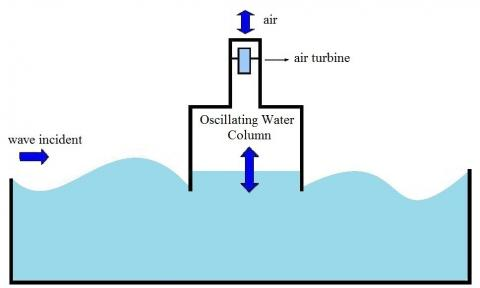Figure 1. Oscillating Water Column Device

2.1 Computational Domain

Figure 2 shows the computational domain used in the present work. The dimensions of the tank (length CT and height HT) are defined taking into account the period (T), height (H) and propagation depth (h) of the incident waves. To avoid reflection, it was considered the length of the tank as five times the wave length; and the wave tank height was the propagation depth plus three times the wave height. Therefore all the characteristic dimensions of the problem are presented in Tab. 1.

2.2 Boundary conditions

As can be observed in Fig. 2, the wave maker is placed in the left side of the wave tank. For the regular wave generation it was employed the called Function . This methodology consists of applying the horizontal (u) and vertical (w) components of wave velocity as boundary conditions (velocity inlet) of the computational model, by means a User Defined Function (UDF) in the FLUENT® software. These velocity components vary as functions of space and time and are based on the Linear Wave Theory. So these wave velocity components are given by:

$u=\frac{H}{2} g k \frac{\cosh (k z+k h)}{\operatorname{woosh}(k h)} \cos (k x-w t)$     (1)

$w=\frac{H}{2} g k \frac{\operatorname{senh}(k z+k h)}{\operatorname{woosh}(k h)} \operatorname{sen}(k x-w t)$       (2)

## 2.pngFigure 2. OWC device and Computational domain

where: H is the wave height (m); g is the gravitational acceleration (m/s2); λ is the wave length (m);  k is the wave number, given by k = 2 π/λ (m-1); h is the depth (m); T is the wave period (s); ω is the frequency, given by ω = 2π/T (rad/s); x is the streamwise coordinate (m); t is the time (s); and z is the normal coordinate (m).

Concerning the other boundary conditions, in the upper surfaces of wave tank and chimney and above the wave maker (dashed line in Fig. 2) it was considered the atmospheric pressure (pressure outlet). In the bottom and right side of computational domain a no slip and impermeability conditions (wall) were adopted.

Table 1. Dimensions of the tank and characteristics of the incident wave

 Dimensions Values Wave Period (T) 5 s Wave Lenght (ʎ) 37.6 m Wave Height (H) 1 m Wave Depht (h) 10 m Tank Lenght (CT) 188 m Tank Height (HT) 13 m
3. Constructal Design

Constructal Design is based on the Constructal Law, stated by Adrian Bejan as follows: “For a finite-size system to persist in time (to live), it must evolve in such a way that it provides easier access to the imposed currents that flow through it”. Thus, the Constructal Design is a method used to find optimal geometric shapes that improve fluid flow and maximize system performance .

Basically, to apply the Constructal Design for the geometric optimization of a physical system it is required an objective function (a quantity that will be optimized), degrees of freedom (geometric parameters which may vary during the optimization process) and geometric constraints (parameters that are kept constant during the optimization process).

So, it was defined as the objective function, the maximization of the hydropneumatic power; as degrees of freedom: H3, H2/l and H1/L and as geometric constraints: the area of the OWC chamber A1 and the total area of OWC device A2, which are given by:

$A_{1}=H_{1} L$     (3)

$A_{2}=H_{1} L+H_{2} l$   (4)

So, from Eq. (3) and Eq. (4) the area of the OWC chamber A1 is equal 37.6 m2 and the total area of OWC device Cis 53.71 m2, respectively. It is worth to mention that areas A1 and A2 are kept constant during application of the Constructal Design method.

Besides Eq. (3) and Eq. (4) are the basis to obtain the values of H1/L and H2/l to analyze the influence of the geometry in the hydropneumatic power. Table 2 presents the values of H1/L and the dimensions of the OWC chamber, and Tab. 3 presents the values of H2/l and the dimensions of the OWC chimney.

Also, to find an optimal submergence for the OWC device, H3 were varied for the values: 10.25 m, 10.00 m, 9.75 m, 9.5 m, 9.25 m, 9.00 m. Both DoFs (H1/L and H2/l) were evaluated for each fixed value of H3.

## 3.png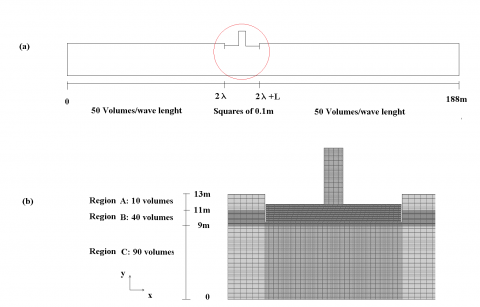Figure 3 (a). Computational domain discretization; (b) Specific area of the OWC converter

Table 2. Dimensions of H1 and L

 Case H1/L L [m] H1 [m] 1 0.0226 37.5969 1.0000 2 0.0399 30.6978 1.2248 3 0.0598 25.0646 1.5001 4 0.0897 20.4652 1.8372 5 0.1346 16.7100 2.2500 6 0.2019 13.6434 2.7558 7 0.3029 11.1398 3.3752 8 0.4544 9.0956 4.1338 9 0.6817 7.4265 5.0629 10 1.0225 6.0637 6.2007

Table 3. Dimensions of H2 and l.

 Case H2/ l l [m] H2 [m] 1 2.3 2.6469 6.0879 2 2.5 2.5388 6.3471 3 3.0 2.3176 6.9529 4 4.0 2.0071 8.0285 5 6.0 1.6388 9.8328
4. Mathematical and Numerical Models

The VOF method is a multiphase numerical model that can be used to treat the interaction between water and air inside the wave tank. In this method, the free surface can be identified by the volume fraction (f) variable. In each mesh cell (volume), if f = 1 the cell is full of water, when f = 0 the cell contain only air and if 0 < f < 1 the cell has water and air simultaneously. Moreover, when the VOF method is used a single set of momentum and continuity equations is applied to all fluids, and the volume fraction of each fluid in every computational cell (control volume) is tracked throughout the domain by the addition of a transport equation for the volume fraction. Thus, the model is composed by the continuity, momentum and volume fraction equations, which are respectively given by [18, 19]:

$\frac{\partial \rho}{\partial t}+\nabla \cdot(\rho \vec{v})=0$     (5)

$\frac{\partial}{\partial t}(\rho \vec{v})+\nabla \cdot(\rho \vec{v} \vec{v})=_{-} \nabla P+\nabla \cdot(\bar{\tau})+\rho \vec{g}$   (6)

$\frac{\partial f}{\partial t}+\nabla \cdot(f \vec{v})=0$     (7)

being: ρ the fluid density (kg/m3), t the time (s), $\vec{v}$ the flow velocity vector (m/s), P the static pressure (Pa), $\overline{\bar{\tau}}$ the stress tensor (Pa) and $\overline{\bar{\tau}}$ the gravitational acceleration (m/s2).

The solver is pressure-based and all simulations were carried out by upwind and PRESTO for spatial discretizations of momentum and pressure, respectively. The velocity-pressure coupling is performed by the PISO method, while the GEO-RECONSTRUCTION method is employed to tackle with the volumetric fraction. Moreover, under-relaxation factors of 0.3 and 0.7 are imposed for the conservation equations of continuity and momentum, respectively. All numerical simulations were carried out in a computer AMD Athlon 2 Core with 3.0Gb of RAM. To reduce the simulation time the parallel processing technique was adopted .

5. Results and Discussions

Computational modeling using the VOF method has been largely employed to numerically simulate the WECs. Validations and verifications of these methodologies can be found in [20-26].

In this work the computational model verification was performed comparing the transient water free surface elevation in a specific position numerically obtained with the respective analytical solution, which is defined by:

$n=A \cos (k x-w t)$     (8)

where: A is the wave amplitude (m), given by H/2.

In Fig. 4 can be observed the numerical and the analytical solutions. The comparison of them was carried out at a stable time interval between 15 and 30 s (where the numerical wave is already adequately formed and there is still no reflection effects associated). The relative difference was measured instantaneously, and the average of these differences was 1.47%, where the minimum value obtained was 0.00104% and the maximum 3.76%. These results show the accuracy of the computational model.

## 4.pngFigure 4. Computational model verification at x = 48 m

To analyze numerically the influence of the geometry of the OWC device, the hydropneumatic power was calculated as follows :

$P_{h y d}=\left(P_{a i r}+\frac{\rho_{a i r} v_{a i r}^{2}}{2}\right) \frac{\dot{m}}{p_{a i r}}$      (9)

where $P_{\text {air}}$ is the static pressure in the OWC chimney (Pa),$P_{\text {air}}$ is the air density (kg/m3), $\dot{m}$

is the air mass flow rate crossing the chimney (kg/s), $v_{a i r}$ is the air velocity in the chimney (m/s) given by:

$v_{a i r}=\frac{m}{A \rho_{a i r}}$   (10)

where A the cross sectional area of the chimney (m2).

For each case, besides the hydropneumatic power, it was evaluated the mass flow rate at the chimney outlet (measured in the computational domain using a monitor in Ansys Fluent®) and the pressure inside the chamber. This pressure was calculated as follows:

$P_{a i r_{-} t}=P_{a i r_{-} e}+P_{a i r_{-}d}$    (11)

where

$P_{a i r_{-} t}$ is the total pressure inside the chamber, $P_{a i r_{-}e}$ is the static pressure measured in Ansys Fluent®, and $P_{a i r_{-} d}$ is the dynamic pressure given by:

$P_{a i r_{-} d}=\frac{1}{2} \rho_{a i r} v_{a i r}^{2}$    (12)

The head loss caused by the turbine was not taken into account in this work.

It was calculated the average values of the hydropneumatic power, the mass flow rate and the pressure using the RMS statistical technique :

$X=\sqrt{\frac{1}{T} \int_{0}^{T} x^{2} d t}$    (13)

The first degrees of freedom evaluated were H1/L and H2/l. Figure 5 shows the results for the hydropneumatic power against H1/L for a fixed value of H3 (9.5 m). Each curve represents a different value of H2/l. It is noticeable there is a value of H1/L that maximizes the hydropneumatic power and it is not altered by H2/l. This value is equal 0.135 which means 16.71 m for the length of OWC chamber L and 2.25 m for its height H1 (the same value was obtained previously in Gomes et al., 2013). So, as declared the dimensions are related to the wave length, Lo (optimal L) represents 0.44 times λ.

## 5.png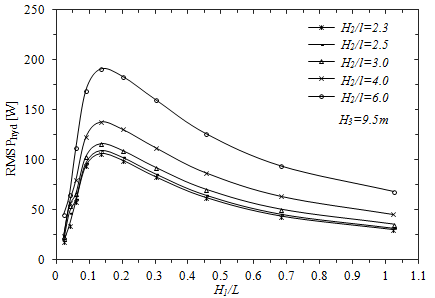Figure 5. RMS hydropneumatic power variation related to H1/L

On the other hand, it is worth to mention that there is an important and expected tendency that can be seen in fig. 5, for higher values of H2/l, it can be obtained higher values of hydropneumatic power. It occurs because the increase of H2/l which is caused by a reduction of the length of the chimney l, causes the increase of the pressure of the air and in consequence the increase of power through the chimney but this fact is going to continue until the dimension of l allows the airflow. As it was shown in , the reduction of l increased the pressure and the hydropneumatic power until l was so short that the airflow was obstructed. This phenomenon occurred for a value of H2/l near to 300, that is, when the height H2 and the length l of the chimney were 69.5 m and 0.2 m respectively, but these dimensions are not viable for the geometry of the chimney of an OWC device, so it was suggested that the optimal value of H2/l is 6.0.

The optimization of ratio H1/L also increases the air mass flow through the chamber.  Figure 6 shows the transient behavior of air mass flow rate through time for the best and worst cases simulated for fixed values of the other DoFs. (H2/l = 6.0 and H3 = 9.5 m). The RMS values for both cases in the stable period between 15 s and 30 s were 15.2 kg/s and 6.4 kg/s respectively. It represents an increase of 42% of air mass flow rate.

Figure 7 shows the transient behavior of air pressure through time for the best and the worst values of H2/l evaluated for fixed values of the other DoFs. (H1/L = 0.135 and H3 = 9.5 m). The RMS values for both cases in the stable period between 15 s and 30 s were 58.3 Pa and 22.4 Pa respectively. It represents an improvement of 39% of air pressure.

Now in Fig. 8 is shown the maximum power obtained (Phyd,o) for each H2/l variation and H3= 9.5 m. Also in Fig. 8 there is a curve that represents the optimal value of H1/L which maximizes the hydropneumatic power for each variation of H2/l. Particularly it is the same value for all cases (H1/L = 0.135) because the optimization of ratio H2/l does not change the air mass flow instead it increases the pressure of the air.

## 6.pngFigure 6. Air mass flow rate behavior for the best and worst values of H1/L

## 7.png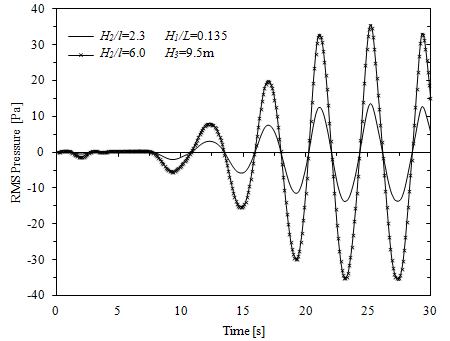Figure 7. Pressure vs time for the best and the worst value of H2/l

## 8.png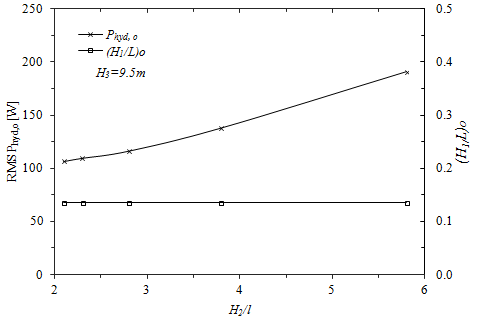Figure 8. Maximum power and optimal H1/L related to H2/l

## 9.jpgFigure 9. Velocity vectors for the sixth period. Right column H2/l = 2.3; Left column H2/l = 6.0

## 10.png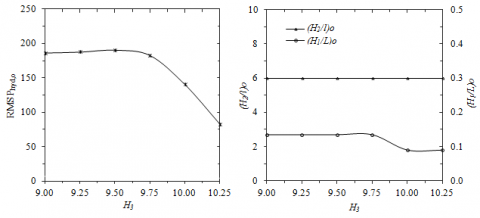Figure 10. (a) Maximum power related to H3; (b) optimal H1/L and H2/l related to H3.

Besides, the reduction of l improves the airflow through the chimney as can be seen in Fig. 9 where it is presented the velocity vectors for the sixth period of wave propagation, for the geometries with the worst (H2/l = 2.3) and the best (H2/l = 6.0) results of the hydropneumatic power. The optimization of H2/l increases the velocity of the air, reduces its recirculation and the airflow becomes more regular.

Now the third degree of freedom is not related with the device’s geometry instead its submergence. The variation of the third degree of freedom H3 (submergence), is treated in Figs. 10 (a) and (b). The maximum hydropneumatic power (Phyd,o)  obtained for each H3 value evaluated is shown in Fig. 10 (a), and the associated values of H1/L and H2/l are in Fig. 10 (b). It can be seen that the maximum hydropneumatic power increases when the device is more submerged (for H3 values less than10 m). Specifically the best performance of the device was between 9.0 m < H3 < 9.75 m which means (h- H) < H3< (h- H/4) in terms of wave depth h and wave height H. This fact is so important because water free surface is not constant and this range means that the device will operate with high efficiency for multiple conditions.

At the same time in Fig. 10 (b) is shown the corresponding values for H2/l and H1/L which allowed to obtain the maximum power for each value of H3 analyzed. It is noticeable that the optimal value of H2/l is the same for all H3 values evaluated (H2/l = 6.0); it means the dimensions of the chimney are not dependent of the submergence. On the other hand, there is more than one optimal H1/L value; however the optimal H1/L for the cases with the highest maximum power obtained was 0.135. In consequence, as a result of the optimization of three degrees of freedom with Constructal Design, the present study can provide the best performance for an OWC device for a wave climate characterized by 37.6 m of wave length and period equal 5 s. The highest hydropneumatic power was 190.8 W for ratios H1/L = 0.135, H2/l = 6.0 and H3 = 9.5 m.

It was demonstrated that it is possible to improve the system performance by modifying the dimensions of the OWC device based on Constructal Design. Furthermore, the results show that the optimal dimensions for the device may be related to the wave climate of a place to maximize the values of air mass flow rate, air pressure and hydropneumatic power. Therefore Constructal Design proves once more to be a very useful tool in the theoretical guidance for the construction of OWC converters.

6. Conclusion

Computational Fluid Dynamics and Constructal Design were used to analyze the dimensions and location in water depth of the OWC-WEC device that allow an optimal performance by maximizing the hydropneumatic power. The conservation equations of mass and momentum for the mixture of water and air, as well as, one transport equation for the volume fraction of water were solved with the Finite Volume Method (FVM). To tackle with the mixture of two phases (water and air) the method Volume of Fluid was employed. Concerning the geometry evaluation, three DoFs were varied (H1/L, H2/l and H3) for fixed areas of the chamber and the device.

The optimal ratio H1/L that maximizes the hydropneumatic power was 0.135 which means L $\cong$ 7.7H1 and L = 0.5λ. On the other hand, the ratio H2/l with best results for the hydropneumatic power was 6.0. It represents H2= 0.26λ. Finally the best performance of the device with the H3 variation was obtained in the range 9.0 m < H3 < 9.75 m which means (h- H) < H3< (h- H/4).

The results also showed that there is a maximum hydropneumatic power around 190 W when optimal ratios H1/L, H2/l and H3 were equal to 0.135, 6.0 and 9.5 m respectively. On the opposite, the worst shape led to an hydropneumatic power of nearly 11 W, showing that the geometry rationalization is also an important subject for the use of renewable energy sources.

It should be noted that a redistribution of the geometry based on Constructal Design can provide a better performance of the device. Besides, the dimensions of the device were related to the wave climate with the purpose of this computational model and Constructal method can be used to supply theoretical information for the construction of the OWC-WEC prototype to take advantage of the wave energy potential at any local with appropriated wave climate.

Acknowledgement

The authors thank to Universidade Federal do Rio Grande do Sul (UFRGS), Universidade Federal de Rio Grande (FURG), and CAPES for the financial support.

References

1. International Energy Agency IEA, http://www.iea.org/topics/renewables/.

2. European Renewable Energy Council (EREC), Renewable Energy in Europe, 2nd ed., Earthscan, UK and USA, 2010.

3. J. M. B. P. Cruz, A. J. N. A. Sarmento, Energia das Ondas: Introdução aos Aspectos Tecnológicos, Económicos e Ambientais, Ed. Instituto do Ambiente, Amadora, Portugal, 2004.

4. A. Bejan, Shape and Structure: From Engineering to Nature, Cambridge University Press, Cambridge, UK, 2000.

5. A. Bejan and S. Lorente, Design with Constructal Theory, Wiley, Hoboken, 2008.

6. A. Bejan and J. Zane, Design in Nature, Doubleday, USA, 2012.

7. A. Bejan and S. Lorente, Constructal Law of Design and Evolution: Physics, Biology, Technology, and Society. Journal of Applied Physics. Vol. 113, pp.1-20, 2013.

8. N. da R. Lopes, F. S. P. Sant'anna, M. N. Gomes, J. A. Souza, P. R. de F. Teixeira, E. D. Dos Santos, L. A. Isoldi, L. A. O. Rocha, Constructal Design Optimization of the Geometry of an Oscillating Water Column Wave Energy Converter (OWC-WEC), Proc. Constructal Law Conference, Federal University of Rio Grande do Sul, Porto Alegre, RS, Brazil, 2012.

9. E. D. Dos Santos, B. N. Machado, N. Lopes, J. A. Souza, P. R. de F. Teixeira, M. N. Gomes, L. A. Isoldi, L. A. O. Rocha, Constructal Design of Wave Energy Converters, Constructal Law and the Unifying the Principle of Design, L. A. O. Rocha, S. Lorent, A. Bejan, Springer, New York, USA, 2013.

10. B. N. Machado, M. M. Zanella, M. N. Gomes, P. R. de F. Teixeira, L. A. Isoldi, E. D. Dos Santos, L. A. O. Rocha, Constructal Design of an Overtopping Wave Energy Converter, Proc. Constructal Law Conference, Federal University of Rio Grande do Sul, Porto Alegre, RS, Brazil, 2012.

11. M. N. Gomes, C. D. Nascimento, B. L. Bonafini, E. D. Dos Santos, L. A. Isoldi, L. A. O. Rocha, Two-Dimensional Geometric Optimization of an Oscillating Water Column Converter in Laboratory Scale. Thermal Engineering, Vol. 11, pp. 30-36, 2012.

12. M. N. Gomes, E. D. Dos Santos, L. A. Isoldi, L. A. O. Rocha, Two-Dimensional Geometric Optimization of an Oscillating Water Column Converter of Real Scale, 22nd International Congress of Mechanical Engineering (COBEM 2013), Ribeirão Preto, SP, Brazil, 2013.

13. M. N. Gomes, E. D. Dos Santos, L. A. Isoldi, L. A. O. Rocha, Two-Dimensional Geometric Optimization of an Oscillating Water Column Converter of Real Scale, 22nd International Congress of Mechanical Engineering (COBEM 2013), Ribeirão Preto, SP, Brazil, 2013.

14. M. F. Espinel, M. N. Gomes, L. A. O. Rocha, E. D. Dos Santos, L. A. Isoldi, Modelagem Computacional e Otimização da Conversão da Energia com Constructal Design de um Dispositivo do Tipo Coluna de Água Oscilante, XXXV Iberian Latin-American Congress on Computational Methods in Engineering CILAMCE 2014, Fortaleza, CE, Brazil, 2014.

15. H. K. Springerverlag Versteeg, W. Malalasekera, An Introduction to Computational Fluid Dynamics – The Finite Volume Method, Pearson, England, 2007.

16. ANSYS Inc., Softwares FLUENT and ICEM, 2013.

17. C. W. Hirt, B. D. Nichols, Volume of Fuid (VOF) Method for the Dynamics of Free Boundaries, J. Comput. Phys., vol. 39, no. 1, pp. 201–225, 1981.

18. Ansys Fluent, Theory Guide, 2009.

19. M. N. Gomes, C. R. Olinto, L. A. O. Rocha, J. A. Souza, L. A. Isoldi, Computational Modeling of a Regular Wave Tank. Thermal Engineering, Vol. 8, pp. 44-50, 2009.

20. T. G. Barreiro, Estudo da interação de uma onda monocromática com um conversor de energia. Dissertação de Mestrado em Engenharia Mecânica, Faculdade de Ciências e Tecnologia da Universidade Nova de Lisboa, 2009.

21. M. Horko, CFD Optimisation of an Oscillating Water Column Energy Converter, MSc. thesis, Mechanical Engineering School, Western University, Australia, 2007.

22. Z. Liu, B. Hyun, K. Hong, Application of numerical wave tank to OWC air chamber for wave energy conversion. International Offshore and Polar Engineering Conference, 2008.

23. Z. Liu, B. Hyun, J. Jin, Numerical prediction for overtopping performance of OWEC. Journal of the Korean Society for Marine Environmental Engineering, Vol. 11, No.1, pp. 35-41, 2008.

24. M. N. Gomes, Modelagem Computacional de um Dispositivo Coluna d'Água Oscilante de Conversão de Energia das Ondas do Mar em Energia Elétrica. MSc, thesis, Universidade Federal do Rio Grande, Rio Grande, RS, Brazil, 2010.

25. R. dos S. Ramalhais, Estudo Numérico de um Dispositivo de Conversão da Energia das Ondas do Tipo Coluna de Água Oscilante (CAO)). Msc. thesis, Faculdade de Ciências e Tecnologia da Universidade Nova de Lisboa, Lisbon, Portugal, 2011.

26. Z. Liu, B. Hyun, K. Hong, Numerical Study of air chamber for oscillating water column wave energy convertor. China Ocean Eng., Vol. 25, pp. 169-178, 2011.

27. N. Dizadji, S. E. Sajadian, Modeling and optimization of the chamber of OWC system, Energy, Vol. 36, pp. 2360– 2366, 2011.

28. A. E. Marjani, F. Castro, M. Bahaji, B. Filali, 3D unsteady flow simulation in an OWC wave converter plant. In Proceedings of the International Conference on Renewable Energy and Power Quality, Majorca, Spain, 2006.